# Module/guidance vector field

Jump to: navigation, search

## Introduction

We have written an algorithm for solving the problem of tracking smooth curves by an unmanned aerial vehicle travelling with a constant airspeed and under a wind disturbance. The algorithm is based on the idea of following a guiding vector field which is constructed from the implicit function that describes the desired (possibly time-varying) trajectory.

For fixed-wings the output of the algorithm can be directly expressed in terms of the bank angle of the UAV in order to achieve coordinated turns. Furthermore, the algorithm can be tuned offline such that physical constraints of the UAV, e.g., the maximum bank angle, will not be violated in a neighborhood of the desired trajectory.

The implementation for rotorcraft is still in the TO DO list.

This work is based on the paper Guidance algorithm for smooth trajectory tracking of a fixed wing UAV flying in wind flows, presented at ICRA 2017. A more rigorous and detailed analysis can be found in the paper A guiding vector field algorithm for path following control of nonholonomic mobile robots.

We can further exploit the convergence properties of the fixed-wing aircraft to the desired trajectory for controlling formations of several aircraft. Check the subsection python Apps. A detailed analysis can be found in the paper Circular formation control of fixed-wing UAVs with constant speeds (submitted to IROS 2017). A video about how the circular formation algorithm works https://www.youtube.com/watch?v=q4b8JRU1Gbw .

Here we show an animation of an airplane following an ellipse and a sinudoidal track.

## How does the algorithm work?

We start from the implicit equation of the trajectory, for example for a circumference we have that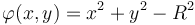$\varphi(x,y) = x^2 + y^2 - R^2$, where$x, y$ and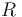$R$ are the x, y coordinates with respect to HOME (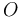$O$ in the following figure) and R is the radius of the circumference. Note that when the vehicle is over the desired trajectory, then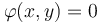$\varphi(x,y) = 0$, otherwise it will be different from zero. We will use this concept of "level set" to define the notion of distance to the trajectory, namely$e := \varphi(x,y)$. It has to be noted that this is different from the Euclidean distance (see figure below).

For the sake of clarity we will consider just 2D trajectories and parallel to the ground. Let us stack the x and y coordinates in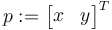$p := \begin{bmatrix}x & y\end{bmatrix}^T$ and consider the following kinematical model for our vehicle$\begin{cases} \dot p &= sm(\psi) + w \\ \dot\psi &= u, \end{cases}$

where$s\in\mathbb{R}^+$ is a constant that can be considered as the airspeed,$m = \begin{bmatrix}\cos(\psi) & \sin(\psi)\end{bmatrix}^T$ with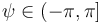$\psi\in(-\pi, \pi]$ being the attitude yaw angle,$w\in\mathbb{R}^2$ is a constant with respect to$O$ representing the wind and$u$ is the control action that will make the vehicle to turn. We also notice that the course heading$\chi\in(-\pi, \pi]$, i.e. the direction the velocity vector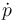$\dot p$ is pointing at, in general is different from the yaw angle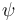$\psi$ because of the wind.

Given a desired trajectory, we compute its normal$n(p) := \nabla \varphi(p)$ and its tangent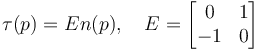$\tau(p) = En(p), \quad E=\begin{bmatrix}0 & 1 \\ -1 & 0\end{bmatrix}$. The idea is to steer the vehicle such that it follows the direction given by the unit vector calculated from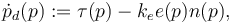$\dot p_d(p) := \tau(p) - k_e e(p)n(p),$

where$k_e$ is a positive gain. At each point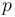$p$ we can build a unit vector from$\dot p_d(p)$. This collection of vectors is our Guidance Vector Field. Note that when the error is zero, then we are just tracking the tangent to the trajectory.

We assume that the trajectory is twice differentiable (so its gradient and Hessian exists) and that it is regular in a neighborhood of$\mathcal{P}$, i.e.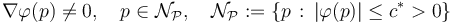$\nabla\varphi(p) \neq 0, \quad p\in \mathcal{N}_{\mathcal{P}},\quad \mathcal{N}_{\mathcal{P}} := \{p \, : \, |\varphi(p)| \leq c^* > 0\}$.

The algorithm needs for work the measurements of$\dot p$ (typically derived from GPS), position$p$ w.r.t. HOME (typically derived from GPS) and yaw angle$\psi$ or sideslip angle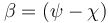$\beta = (\psi - \chi)$. The algorithm still works quite well in practice with only$\dot p$ and$p$, in other words, you will only need to have installed a GPS. The latter is the implemented version in pprz/master.

## Using the GVF in the flight plan

Before starting, make sure that the environment variable "PAPARAZZI_HOME" is set. It must point to your paparazzi directory. To check if this variable is set, enter in a terminal the following command:

One can synchronize or arrange a group of fixed-wing aircraft (assuming they have equal ground speed) in a desired circle by using the python script at "$PAPARAZZI_HOME/sw/ground_segment/python/gvf/gvfFormation". For running the script, all your aircraft MUST follow a circle employing the guidance vector field, i.e., <call fun="gvf_ellipse_wp(WP_CIRCLE, gvf_ellipse_par.a, gvf_ellipse_par.b, gvf_ellipse_par.alpha)"/>  the values of gvf_ellipse_a, gvf_ellipse_b will be modified by the formation control script, and the value of gvf_ellipse_alpha is irrelevant. Note that the aircraft do not need necessarily to orbit around the same waypoint. We need three text files in order to run the script: • ids.txt , which labels the aircrafts. For example, if the file contains '14 56 34', then the aircraft with ids 14, 56 and 34 are labeled with the tags 1, 2 and 3 respectively. • topology.txt , which defines the links between the aircraft. The file contains a matrix where the number of rows is the number of aircraft, and the number of columns is the number of desired links. A link is defined by setting 1 and -1 in a column. Following the example, the matrix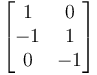$\begin{bmatrix}1 & 0 \\ -1 & 1 \\ 0 & -1\end{bmatrix}$ defines two links. The first one between the aircraft 1 and 2, and the second one between the aircraft 2 and 3. • sigmas.txt , which defines the desired angles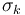$\sigma_k$ between aircraft for each link$k$. Following the example, if the file contains '0 90', then the desired angle for the first link is 0 degrees (e.g., the aircraft 1 and 2 will meet each other) and the second desired angle will be +90 degrees (positive is clock-wise). Some useful tips: • How you place 1 and -1 in the topology matters in the following sense. In our example, for the second link, the +90 degrees is from the aircraft 2 to the aircraft 3. If the topology were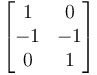$\begin{bmatrix}1 & 0 \\ -1 & -1 \\ 0 & 1\end{bmatrix}$, then the +90 degrees will be from the aircraft 3 to the aircraft 2. • Watch out for "impossible" configurations, e.g., do not set up loops in the topology such as 1 -> 2 -> 3 -> 1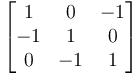$\begin{bmatrix}1 & 0 & -1\\ -1 & 1 & 0 \\ 0 & -1 & 1\end{bmatrix}$, so the desired sigmas could be asking for an impossible configuration in the circle. Avoid loops! even if the desired configuration is ok, they can introduce undesired equilibria in the system, i.e., different configurations. • The aircraft do not need to share the same center or waypoint. This script just control the sigmas. The script needs two additional scalar values: • radius , sets the desired radius of the circular formation, i.e., once the aircraft are synchronized, they will be orbiting the waypoint with this desired radius. • k, this is the gain of the control algorithm. As an example, for three aircraft with a desired circumference of 80 meters, a value of$k=10$ works ok. The algorithm works by setting different radii to the aircraft. If two aircraft orbit a waypoint with different radius (assuming equal ground speeds), then the one with bigger radius travels more distance in a completed lap. We exploit this fact in order to control the different$\sigma_k$. We refer to Circular formation control of fixed-wing UAVs with constant speeds for details about the control signal and how to calculate an appropriated gain k. In the following figure we show the rendezvous of three aircraft in the same circle by using this script. ## Fully distributed circular formations The air-to-air communication is possible since Paparazzi Link v2.0. You can compile Paparazzi with this new feature by typing "PPRZLINK_LIB_VERSION=2.0 make" in your terminal. The algorithm for synchronizing aircraft in a circle has been explained in the previous section "Circular formations (centralized from the GCS)". However, in that section it is explained how to run the algorithm in a centralized way, i.e., every aircraft transmit their positions to the GCS and a python script sends back the radii that the GVF must follow for each aircraft. Now it is possible to let each aircraft to calculate on board the radius that it has to follow by exchanging information with its neighbors without any intervention from ground. An extra module together with gvf_module has to be called in the flight plan <modules> <module name="gvf_module"/> <module name="distributed_circular_formation"/> </modules>  and the following settings can be set in the airframe file <section name="DCF" prefix="DCF_"> <define name="GAIN_K" value="10"/> <define name="RADIUS" value="80"/> <define name="MAX_NEIGHBORS" value="4"/> </section>  where GAIN_K is the control gain k explained in the centralized version (note that now each aircraft can have its own different gain), RADIUS is the target radius for the desired circumference once the synchronization is achieved, and MAX_NEIGHBORS defines the maximum number of neighbors that an aircraft can have. In particular, this last number defines how big is a table stored on board with the information about the neighbors of an aircraft. These three settings can be modified via the DCF tab from the GCS settings menu. Each row of the table contains information about a specific neighbor. In particular there are four fields about the neighbor, its ID, the desired angle w.r.t. it, its actual sigma (IT IS CALLED THETA in the source code dcf.{c,h} and messages! sorry about the notation ;) ) and how old is the actual sigma, i.e., when was the last time the aircraft received information from its neighbor. There is a telemetry message called DCF where one can check the status of the table, where the angles are in deci-degrees, i.e., 900 is 90 degrees and the age of the information is in milliseconds. The following messages must be included in your custom ./conf/messages.xml (if they are not present in your PaparazziLink version yet) <message name="DCF" id="38"> <field name="table" type="int16[]"/> <field name="errors" type="int16[]"/> </message> <message name="DCF_THETA" id="254"> <field name="theta" type="float"/> </message> <message name="DCF_REG_TABLE" id="158" link="forwarded"> <field name="ac_id" type="uint8"/> <field name="nei_id" type="uint8"/> <field name="desired_sigma" type="int16"/> </message> <message name="DCF_CLEAN_TABLE" id="159" link="forwarded"> <field name="ac_id" type="uint8"/> </message>  where the first two messages are msg class telemetry and the last two are msg class datalink. The ids are irrelevant, just look for one free for the messages. The DCF message transmits the table (with low frequency) from the air to the ground. The DCF_THETA is the message that it is transmitted between aircraft. The DCF_REG_TABLE msg inits the table of the aircraft with a particular ID and DCF_CLEAN_TABLE just cleans the table. The python script dcfInitTables.py at ./sw/ground_segment/python/gvf will generate and send the messages based on the same text files for the centralized version of the algorithm (just check the subsection above to see how to define topology, IDs.. etc). DO NOT FORGET to init the tables of the aircraft! You can check that the tables have been well set by checking the corresponding DCF telemetry msg. The algorithm starts running once the aircraft is in the following block in the flight plan <block name="Distributed_circular"> <call fun="distributed_circular(WP_C)"/> </block>  and if the aircraft is in AUTO2, then it starts to transmit its sigma to its neighbors. The aircraft will track (via the GVF) a circumference with center at the waypoint WP_C and the radius is determined by the algorithm. If there are not neighbors, it will just track a circumference with the default radius set in the DCF module (remember that the radius can be also changed in settings in the GCS). Right now, the frequency for the air-to-air communication for the DCF_THETA msg has been hardcoded to 4Hz (something to change in the TODO list). If an aircraft does not receive any information from a neighbor during the last TIMEOUT millisecs, then the aircraft assumes that such a neighbor has left the formation. For example, the neighbor went to AUTO1 and the rest of the team will not consider it anymore. The TIMEOUT has been set to 1500 by default, but it can be changed for each aircraft in the settings in the GCS in the DCF tab. ## Defining your own trajectory The algorithm is placed in$PAPARAZZI_HOME/sw/airborne/modules/guidance/gvf . The trajectories are placed in \$PAPARAZZI_HOME/sw/airborne/modules/guidance/gvf/trajectories .

Here there is an example (the straight line) about how to code your own trajectory.

 File: sw/airborne/modules/guidance/gvf/gvf.h // Straigh line extern bool gvf_line_XY_heading(float x, float y, float heading); extern bool gvf_line_XY1_XY2(float x1, float y1, float x2, float y2); extern bool gvf_line_wp1_wp2(uint8_t wp1, uint8_t wp2); extern bool gvf_segment_loop_XY1_XY2(float x1, float y1, float x2, float y2, float d1, float d2); extern bool gvf_segment_loop_wp1_wp2(uint8_t wp1, uint8_t wp2, float d1, float d2); extern bool gvf_segment_XY1_XY2(float x1, float y1, float x2, float y2); extern bool gvf_segment_wp1_wp2(uint8_t wp1, uint8_t wp2); extern bool gvf_line_wp_heading(uint8_t wp, float heading); 

 File: sw/airborne/modules/guidance/gvf/gvf.c bool gvf_line_wp_heading(uint8_t wp, float heading) { heading = heading * M_PI / 180; float a = waypoints[wp].x; float b = waypoints[wp].y; gvf_line_XY_heading(a, b, heading); return true; } 

The idea is that while you can define a straight line in many different ways, you make all the transformations (for example, we take the X and Y coordinates from a waypoint and convert the heading from degrees to radians in this example) in the function called by the user such that the algorithm is always evaluating the trajectory in the same way. It helps for maintaining the code and for tuning the gains (you do not want to have different set of gains depending on how you call your trajectory).

We finally call the function gvf_line_XY_heading that will invoke the algorithm.

 File: sw/airborne/modules/guidance/gvf/gvf.c bool gvf_line_XY_heading(float a, float b, float heading) { float e; struct gvf_grad grad_line; struct gvf_Hess Hess_line; gvf_trajectory.type = 0; gvf_trajectory.p = a; gvf_trajectory.p = b; gvf_trajectory.p = heading; gvf_line_info(&e, &grad_line, &Hess_line); gvf_control.ke = gvf_line_par.ke; gvf_control_2D(1e-2 * gvf_line_par.ke, gvf_line_par.kn, e, &grad_line, &Hess_line); gvf_control.error = e; horizontal_mode = HORIZONTAL_MODE_WAYPOINT; gvf_segment.seg = 0; return true; } 

The three first lines are the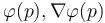$\varphi(p), \nabla\varphi(p)$ and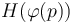$H(\varphi(p))$, where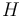$H$ stands for the Hessian. These three guys will be populated by gvf_line_info (in a moment we will be see how to write it).

Then gvf_traj_type stands for the kind of trajectory. Right now we have 0 for lines, 1 for ellipses and 2 for sinusoidals. Feel free to choose an index that has not been taken. The rest gvf_param[x]'s are the parameters of the trajectory. You have up to seven for describing a trajectory.

The function gvf_control_2D executes the algorithm for calculating the desired turn for the vehicle. It is a good practice that all the trajectories (not only straight lines) have the same order of magnitude (for example between 0.00 and 5.00) for$k_e$. So if you have to add a scaling factor, here is were you have to do it.

The variables gvf_xx are sent to the ground station as telemetry, so you can draw the vector field, the trajectory, etc.

Finally for defining$\varphi(p), \nabla\varphi(p)$ and$H(\varphi(p))$ you need to write the two files gvf_line.{c,h}

 File: sw/airborne/modules/guidance/gvf/trajectories/gvf_line.h extern void gvf_line_info(float *phi, struct gvf_grad *, struct gvf_Hess *); 

and in gvf_line.c you write the implicit math of your trajectory (quite explicit)

 File: sw/airborne/modules/guidance/gvf/trajectories/gvf_line.c void gvf_line_info(float *phi, struct gvf_grad *grad, struct gvf_Hess *hess){ struct EnuCoor_f *p = stateGetPositionEnu_f(); float px = p->x; float py = p->y; float a = gvf_param; float b = gvf_param; float alpha = gvf_param; // Phi(x,y) *phi = -(px-a)*cosf(alpha) + (py-b)*sinf(alpha); // grad Phi grad->nx = -cosf(alpha); grad->ny = sinf(alpha); // Hessian Phi hess->H11 = 0; hess->H12 = 0; hess->H21 = 0; hess->H22 = 0; } `

## Demo

There is a flightplan called 'demo_gvf.xml' in order to test the different settings in a simulation. Do not forget to choose default_telemetry_gvf.xml in your paparazzi center.

## TO DO list

• Better integration of the GVF with the ground station
• Support to rotorcrafts